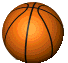GeoCitesSites.com
```Problems```

This is the core of The Vault - logic based programming problems. You'll find a list of problems below, but before that I should tell you that since I've been using QBasic quite a lot, the QBasic syntax may have creeped into some problems. Basically, the \$ sign means a string. So A\$ means a string denoted by A. Besides that there isn't much QBasic interference in the problems.

The problems are given in detail, so if the short description given is vague, be sure to check out the link. Oh, and you can just browse through the problems as well. Click on any of the problem links below, then use the left and right buttons to go to the previous and next one respectively.

Oh, and I must mention, unlike before new problems will not be necessarily added one at a time, or regularly. As and when I get the time and inspiration, I'll put them up.
 # Title Short Description Wilcard matches A simple string manipulation problemm 2 Chains of words Verify if a given set of words can be re-ordered for form a chain 3 The Dollar code A string-manipulation based encryption and decryption problem. The rules of encrypting are given, you just have to apply them. 4 The Hell problem One of me favourites. Give all possible permutations of the letters of a word, but reject duplicates. 5 Rotating Words A really easy string manipulation problem. 6 Shred-Merge Another one of my favourites. See if a given word can be formed by shredding two other words in any possible fashion and then merging the shredded pieces. You have to see this one. 7 Two strings in a spiral Generate a spiral pattern on the screen, using two strings and some other complications. 8 Spiral constellations Generate a simple spiral on the screen. 9 Roman Numbers Convert Indo-Arabic numbers (the numbers we use) to Roman Numbers. The values of Roman numerals are given. 10 Roman to Indo-Arabic The reverse of the previous problem. Given a Roman number, convert it into the corresponding Indo-Arabic number. 11 The Jackpot Path An interesting problem. The first instinct may be to use recursion, but... 12 Amicable numbers A really simple one. Just tell if two numbers are Amicable or not. 13 The number tree Given some numbesr, fill the empty spaces to complete the tree. 14 Expression Evalutaion Evaluate a given mathematical expression using the standard rules (of operator preference etc.) 15 The Saddle Point Umm.. just read the whole thing. 16 A tree or not a tree Verify if the given nodes and connections form a single tree. 17 The lowest terms Reduce a fraction to it's lowest terns. 18 Die Hard Remember the movie 'Die Hard with a Vengeance' and the water bottles and the bomb? 19 Queens at peace One of the most famous and standard problems in programming history. 20 Key numbers Generate key-numbers using primce factorization. 21 Exponential raise Raise a number to an exponent without using the inbuilt function of your compiler. 22 Shortest Path (Maze) Another famous problem, solve a maze. 23 Russian Peasants An interesting method of multiplication used by Russian peasants, at some point of time. Just follow the rules. 24 Fractions to Decimals Convert a fraction to it's decimal form, and for recurring decimals ... 25 Sharing News You'll have to read the whole thing. 26 The Overlap sequence A modification and extension to the word-chain problem. Involves some text-formatting skills. 27 Coach Select the best possible team of players. 28 Factorials Return the factorial of large numbers (like 56), as well as small numbers! 29 Big-Mult Multiply two large numbers (like ones with 60 digits) 30 The sum of powers A small but nice one. 31 Mustapha's herd Derived from a famous brain teasing story.(Back to The Vault)